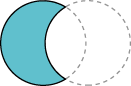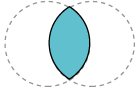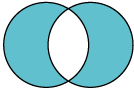Trail: 2D Graphics
Lesson: Advanced Topics in Java2D
Constructing Complex Shapes from Geometry Primitives

# Constructing Complex Shapes from Geometry Primitives

Constructive area geometry (CAG) is the process of creating new geometric shapes by performing boolean operations on existing ones. In the Java 2D™ API the `Area` class implements the `Shape` interface and supports the following boolean operations.UnionSubtractionIntersectionExclusive-or (`XOR`)

## Example: Areas

In this example `Area` objects construct a pear shape from several ellipses.

Note:

If you don't see the applet running, you need to install release 6 (or later) of the Java SE Development Kit (JDK).

`Pear.java` contains the complete code for this applet.

The leaves are each created by performing an intersection on two overlapping circles.

```leaf = new Ellipse2D.Double();
...
leaf1 = new Area(leaf);
leaf2 = new Area(leaf);
...
leaf.setFrame(ew-16, eh-29, 15.0, 15.0);
leaf1 = new Area(leaf);
leaf.setFrame(ew-14, eh-47, 30.0, 30.0);
leaf2 = new Area(leaf);
leaf1.intersect(leaf2);
g2.fill(leaf1);
...
leaf.setFrame(ew+1, eh-29, 15.0, 15.0);
leaf1 = new Area(leaf);
leaf2.intersect(leaf1);
g2.fill(leaf2);
```

Overlapping circles are also used to construct the stem through a subtraction operation.

```stem = new Ellipse2D.Double();
...
stem.setFrame(ew, eh-42, 40.0, 40.0);
st1 = new Area(stem);
stem.setFrame(ew+3, eh-47, 50.0, 50.0);
st2 = new Area(stem);
st1.subtract(st2);
g2.fill(st1);
```

The body of the pear is constructed by performing a union operation on a circle and an oval.

```circle = new Ellipse2D.Double();
oval = new Ellipse2D.Double();
circ = new Area(circle);
ov = new Area(oval);
...
circle.setFrame(ew-25, eh, 50.0, 50.0);
oval.setFrame(ew-19, eh-20, 40.0, 70.0);
circ = new Area(circle);
ov = new Area(oval);